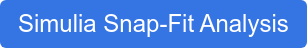SOLIDWORKS Simulation Premium enables you to virtually test real-life scenarios more accurately. It provides you with a wide variety of material models for nonlinear analyses. Elastomers such as rubber, neoprene etc. are materials that go through large deformation very rapidly with relatively a low value of stress or applied load. In this article, we go over the different hyper elasticity material models available in SOLIDWORKS Simulation and how to use them to work with these types of materials.

### Hyper Elasticity

Foam and rubber-like materials can be modeled using any of the three hyper elasticity material models available in SOLIDWORKS Simulation Premium, namely, Mooney-Rivlin, Ogden and Blatz-Ko. These hyper elasticity models assume materials to be nonlinear, elastic, isotropic and incompressible. Poisson’s ratio greater or equal to 0.48, but less than 0.5 is acceptable for hyper elastic materials. Even though the material is treated as elastic, its behavior is rather complex and depends on the loading mode. As opposed to a stress-strain graph, a stress-stretch ratio graph is input for such materials.###### Figure 1: Stress-Stretch Ratio Graph (Click to enlarge)

To define hyper elastic material models such as Mooney Rivlin or Ogden, users can either define constants directly in the properties tab of the material dialog box or provide test data for SOLIDWORKS Simulation Premium to evaluate constants internally.

### Mooney Rivlin (M-R)

This material model can be used with solid elements and shell elements. When custom defining a rubber-like material using M-R model, you can input up to six Mooney Rivlin constants. When inputting these constants directly in the material dialog box, make sure to set the “No. of constants” drop-down menu to appropriate value – 2, 4, 5 or 6, to enter the required number of constants. If the constants are not known, you can enter the test data curve and let the software evaluate them internally.

###### Figure 2: Defining Mooney Rivlin Hyper elastic Material Model in SOLIDWORKS Simulation (Click to enlarge)

Depending upon the available data, it is recommended to provide all three curves - simple tension, planar tension, pure shear or biaxial tension. This data can be obtained from material supplier or testing. M-R material model with two constants (i.e. 2-term) can model strains up to 150%, however, for modeling materials that undergo strains as large as 600%, M-R model with more than 3 (up to 6) constants must be defined.

######Figure 3: Mooney Rivlin hyper elastic material model with six constants in SOLIDWORKS Simulation (Click to enlarge)

Young’s modulus E is not required to be input, however, in the absence of experimental information, the first two constants for a 2-term M-R model may be approximated as:
First material Constant A (or C10) = 0.8E and second material constant B (or C01) = 0.2E. Constants A and B must be defined such that summation of A and B is greater than 0.

### Ogden Hyper Elastic Material Model

Ogden hyper elastic can be used to model rubber-like materials undergoing large deformations. A 3-term Ogden material model can describe deformations for strains up to 600%. Depending upon the number of constants or terms selected in the material drop down menu, you need to enter the power coefficients, Alpha and the Ogden material constants Mu.
>> Read our Product Story: SOLIDWORKS Helps ADMET Push the Limits of Simulated Material Testing

Only as many power coefficients and Ogden material constants are to be input as the number of constants selected in the drop-down menu.

######Figure 4: Ogden hyperelastic material model with six constants in SOLIDWORKS Simulation (Click to enlarge)

If the test data curve is provided, the software will calculate the constants, and this can be found in the solver messages window found by right clicking on the result folder in the study manager. ASCII text files in the result directory, *.LOG, *.LAG and *.PLT contain the material model data. The *.PLT contains a table that compares stress values computed by the software with the stresses input into the software from test data for different stretch ratio values.###### Figure 5: Mooney Rivlin material constants computed in SOLIDWORKS Simulation Premium (Click to enlarge)

SOLIDWORKS Simulation uses the displacement/pressure formulation technique for M-R and Ogden material models as it can resolve volumetric locking when the material is nearly incompressible.

### Hyper Elastic Blatz-Ko

This model can be used for compressible polyurethane foam type rubbers. Elastic modulus, tensile strength and mass density can be input for this material model. Poisson’s ratio of 0.25 is used as the default value by the software to calculate bulk modulus. This model is only supported for solid elements. To model foam in compression, you could also use nonlinear elastic material type as that can allows for a nonlinear curve in compression.

Below are few recommendations to consider when using hyper plastic materials:

• Displacement or Arc-length control method may be more effective than the force control method due to the highly nonlinear nature of the problem.
• Use small value for initial time step and ramp up the load slowly. A rapid increase in loading will often result in numerical instability or divergence during equilibrium iterations.
• Enable the auto-stepping option so the software can automatically adjust the load increments for each solution step to improve chances of convergence. Newton-Raphson iterative method is recommended.
• Poisson’s ratio greater than 0.48 but less than 0.5 (0.4995) is acceptable.
######Displacement Plot of an O-ring rubber seal using SOLIDWORKS Simulation Premium (Click to enlarge)

SOLIDWORKS Simulation Premium, with such advanced material models, allows for realistic virtual testing quickly and accurately. If you run into convergence issues for highly nonlinear problems, checkout Simulia’s SSE, which is a natural extension of SOLIDWORKS Simulation.